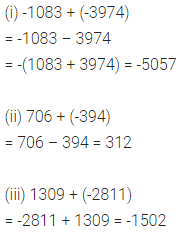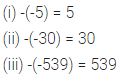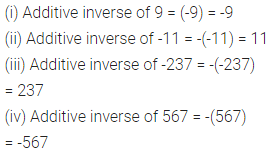# ML Aggarwal Class 6 Solutions for ICSE Maths Chapter 3 Integers Ex 3.2

## ML Aggarwal Class 6 Solutions for ICSE Maths Chapter 3 Integers Ex 3.2

Question 1.
Evaluate the following, using the numbers line
(i) 4 + (-5)
(ii) (-4) + 5
(iii) 7 + (-3)
(iv) -6 + (-2)
Solution:Question 2.
Evaluate the following :
(i) (-8) + (-14)
(ii) -35 + (-47)
(iii) 91 + (-48)
(iv) (-203) + 501
(v) (-36) + 29
(vi) (-131) + 97.
Solution:Question 3.
Evaluate the following :
(i) -1083 + (-3974)
(ii) 706 + (-394)
(iii) 1309 + (-2811)
Solution:Question 4.
Fill in the following blanks :
(i) -(-5) = ……..
(ii) -(-30) = …….
(iii) -(-539) = ………
Solution:Question 5.
Write down the additive inverses of:
(i) 9
(ii) -11
(iii) -237
(iv) 567
Solution: